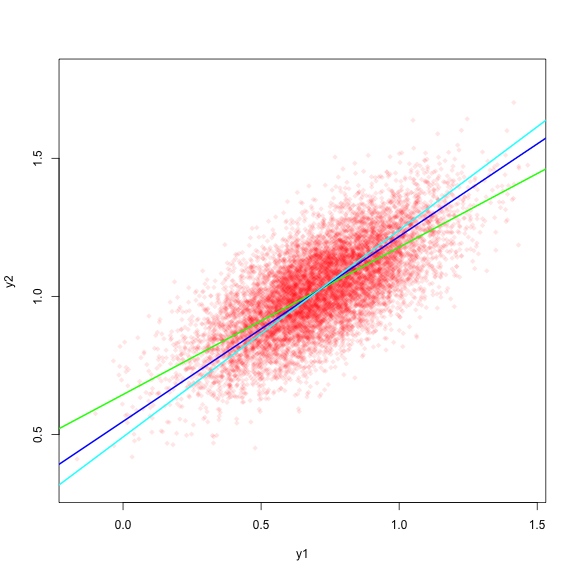# Comparing RS products using model II regression

Assume our surface variable (i.e. LAI) follows a normal distribution:

xv <- rnorm(10000,0.971,0.158)

and that we have a former product (i.e. MODIS MOD**) that linearly estimates x

e <- rnorm(length(xv),0,0.1)
y1 <- xv*1.25 - 0.5
y1 <- y1 + e

and furthermore that we have an improved product (i.e. GEOV3 LAI) that also estimates LAI linearly, with less bias and random error

e <- rnorm(length(xv),0,0.1)
y2 <- xv*0.85 + 0.2
y2 <- y2 + e

Now, let’s apply 3 methods to calculate the correlation between products y1 and y2

lmod <- lmodel2(y2~y1, nperm=99)
print(lmod)
##
## Model II regression
##
## Call: lmodel2(formula = y2 ~ y1, nperm = 99)
##
## n = 10000   r = 0.7123515   r-square = 0.5074446
## Parametric P-values:   2-tailed = 0    1-tailed = 0
## Angle between the two OLS regression lines = 18.35553 degrees
##
## Permutation tests of OLS, MA, RMA slopes: 1-tailed, tail corresponding to sign
## A permutation test of r is equivalent to a permutation test of the OLS slope
## P-perm for SMA = NA because the SMA slope cannot be tested
##
## Regression results
##   Method Intercept     Slope Angle (degrees) P-perm (1-tailed)
## 1    OLS 0.6444808 0.5336780        28.08786              0.01
## 2     MA 0.5470365 0.6701379        33.82754              0.01
## 3    SMA 0.4905951 0.7491779        36.83974                NA
##
## Confidence intervals
##   Method 2.5%-Intercept 97.5%-Intercept 2.5%-Slope 97.5%-Slope
## 1    OLS      0.6367749       0.6521866  0.5233704   0.5439856
## 2     MA      0.5377379       0.5562244  0.6572712   0.6831596
## 3    SMA      0.4831839       0.4979049  0.7389412   0.7595564
##
## Eigenvalues: 0.06648525 0.009972002
##
## H statistic used for computing C.I. of MA: 7.978025e-05

Note the difference between results from OLS and MA (and SMA). By construction, the slope of y2~y1 should be 0.85/1.25 = 0.68. MA and SMA results are much closer to this value than OLS, SMA being more appropriate method when the dispersion of y1 and y2 are different.

OLS <- unlist(lmod$"regression.results"[1,2:3]) MA <- unlist(lmod$"regression.results"[2,2:3])
SMA <- unlist(lmod\$"regression.results"[3,2:3])
plot(y1,y2, pch=18,col=alpha("red",alpha=.1),asp=1)
abline(OLS,col="green",lwd=2)
abline(MA,col="blue",lwd=2)
abline(SMA,col="cyan",lwd=2)plot of chunk unnamed-chunk-6

The lower OLS slope is a consequence of OLS method assuming all the dispersion is due to the dependent variable only, which is not the case when comparing two products. OLS minimizes the squared differences between the observed Y and the predicted Y. MA and SMA take the dispersion in both variables into consideration: MA minimizes the perpendicular distance from the observed point to the predicted line, and SMA minimizes the area of the triangle defined by the observation and the corresponding values on the predicted line.